1. Calculation functions
2. Functions
3. Formula Editing

Here, you can learn how to enter and edit a formula for your line items, how to work with the formula editor, learn about the different levels at which you can set-up a formula, and understand the role of calculation functions in formulas:

Editing a formula is easy in Anaplan. You can:

• Switch between modules or settings when in formula edit mode
• Toggle in and out of Blueprint whilst editing
• See which module is being edited and get there quickly
• Complete a formula
• Exit edit mode easily

When you're working in edit mode the formula editor is always visible, which means that you can continue editing, complete a formula, or exit edit mode at any time—even when you're viewing a different module. No jumping between modules to build your formulas—everything you need is right on screen.

Enter edit mode by clicking in the formula bar when in grid or Blueprint for a module. You'll notice an icon at the top of the page: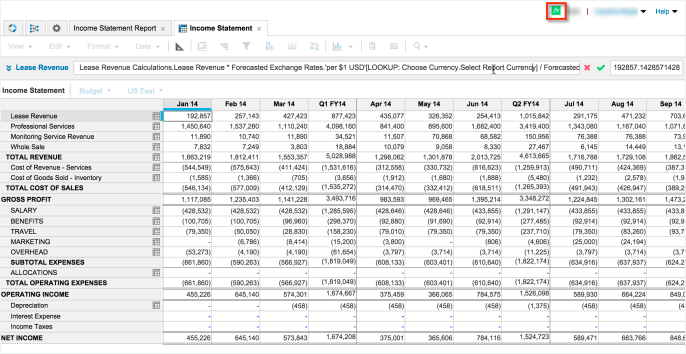The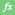icon indicates that you're in formula edit mode. Hover over or click the icon at any time to view more information about the formula you're editing: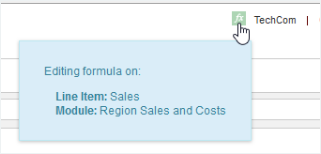The formula editor is always visible, which is great when you're working with multiple modules. You can position the formula editor right where you want it or you can dock it in its original location. It will always stay on top, regardless of where you're working in Anaplan.

## Entering and Editing a Formula

1. Click the name of the line item that you want to apply the formula to, then double-click in the formula bar above the grid. The system switches to formula edit mode and theicon appears at top right and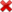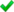appears at the right-side of the formula bar.
2. You can either:
• Type the formula
• Select other line items by clicking their labels in the grid
• Click a data cell (this is equivalent to selecting the line item; Anaplan formulas are expressed in terms of line items, not cells.)
1. Clickor press Enter to apply the formula.
2. If you need more space for viewing or editing a formula, click the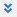icon at the left end of the formula bar. A formula editing panel opens that also has buttons for common operators. See Calculation Functions for the functions that are available.
3. Once you've started to edit a formula, click another tab. The formula editor floats over the page. Grab the header bar and drag the formula editor anywhere on the page.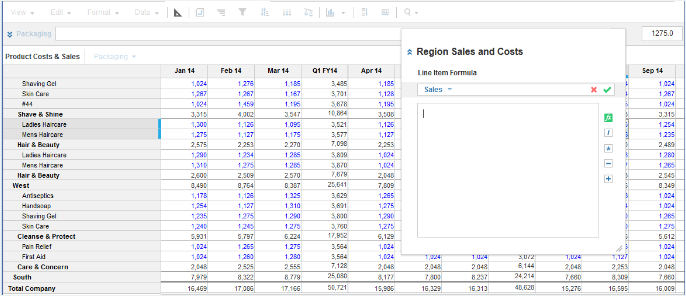The name of the module you're currently editing is displayed at the top of the editor, so you can easily identify where you're working.

You can position the formula editor anywhere you want and Anaplan will remember this position next time you edit a formula. You'll be warned if you try and exit the model while in formula edit mode—you can save your formula or cancel to exit.

Make sure you:

• Close each open parentheses when writing a formula.
• Enter all the required arguments.
• Do not 'nest' more than 100 functions in a single formula.
• Enclose line items name that contain hyphens, numbers or any operator (+ - / *) in single quotes.

## Working with the Formula Editor

If you switch to another tab in formula edit mode, the editor floats on top, allowing you to move around modules. Grab the header bar to drag the formula editor and position it anywhere on the page.

To dock a floating formula editor, click the chevrons at the top right: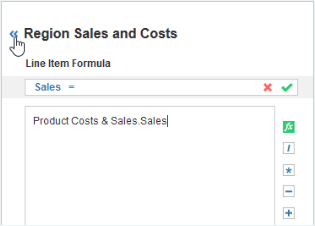The editor will snap back to its original position, which in this case is as a sidebar.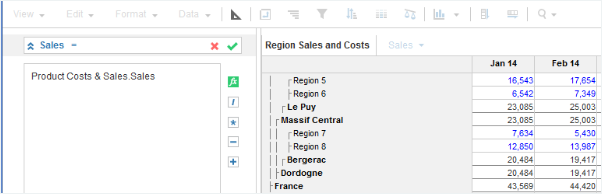In this case, it will snap back horizontally.

If the formula editor is docked, it will float as soon as you switch to another module. To exit edit mode without saving the formula, click. To save and exit, click.

Undocked Modules

You can't float the formula editor if the module you're working in is undocked—the formula edit bar stays docked. And, you can't undock a module that already has a floating formula editor.

## Setting-up formulas at different levels

There are three main ways of setting-up formulas for your line items and these have different levels of application:

1. Individual line item formulas. These apply to a specific line item but you can select to include all or only some of the model versions in the formula scope.
2. Formulas that apply to a specific version for a set of line items within a single module. Each line item you want to apply this type of module scope version formula to must:
• Have versions applied to it.
• Belong to a line item subset.
3. Formulas that are set on a specific version dimension (typically for variance calculations). These also apply to a specific version but their scope of application is the entire model.
• Each line item you want this type of model scope version formula to apply to must have versions applied to it.
• Only numeric line items are affected; non-numeric-formatted line items, such as text-formatted, are left blank.

Which formula level applies to a line item?

When you select a line item, you can read-off the formula and determine which level of formula is applied from the formula bar:

• If an individual line item formula applies, the name of the line item is shown:
•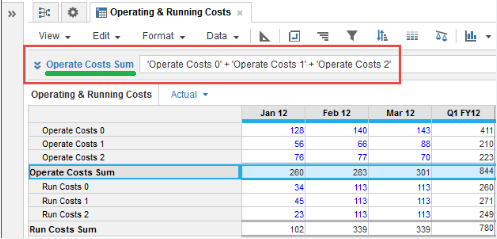• If an individual line item formula applies and you have selected the Formula Scope to be any option other than All Versions, then the versions it applies to is also shown. For example, this formula applies to Current Version:
•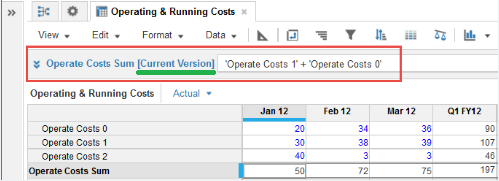• If a module scope version formula applies, the version and line item subset the formula applies to is shown:
•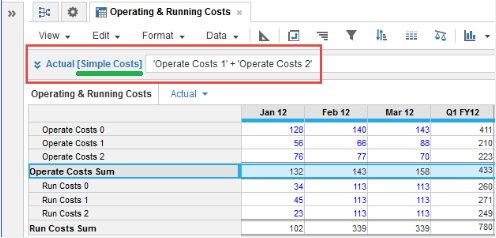• If a model scope version formula applies, the version the formula applies to is shown:
•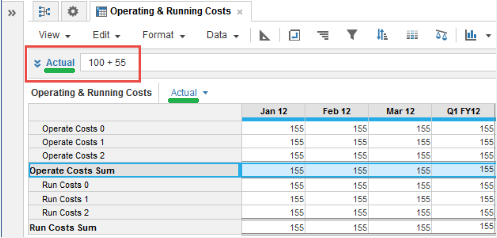• Note that non-numeric-formatted line items are left blank for model scope version formulas—here Month Operating Manager is text-formatted:
•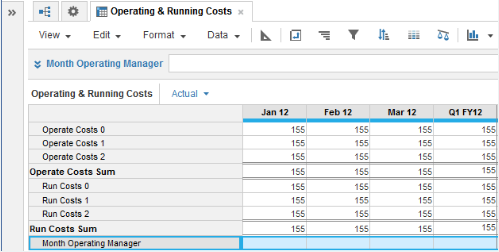Formula Precedence

The order of precedence when you try to enter formula at all three levels that apply to the same line item is as follows:

• If you enter a formula for an individual line item but there is no module scope version formula and you enter a version formula for the entire model, then the version formula for the entire model takes precedence.
• If you enter an individual line item formula and you then try to enter a module scope version formula that also applies to the line item, then you will be blocked—a message appears telling you of the formula clash.
• If a module scope version formula applies to a line item and you try to enter an individual line item formula also for the line item, then you will be blocked—a message appears telling you of the formula clash.
• If module scope version formulas already exists and you try to enter a version formula for the entire model that clashes with an existing module scope version formula, then you will be blocked—a message appears telling you of the formula clash.
• If version formulas for the entire model already exist and you try to enter a module scope version formula that clashes with a version formula for the entire model, then you will be blocked—a message appears telling you of the formula clash.
If you have designated a line item as an Is Summary line item for other line items in the module and used the required simple sum formula for it, this formula takes precedence and can co-exist with a module scope version formula that also applies to the Is Summary line item.

How to enter formula at each level is explained in the following sections:

#### Adding individual line item formulas

Double-click in the formula bar at the top of the tab and then type in the formula. Or, click the items to include and then edit the operators (+ - / *) or one of the available functions.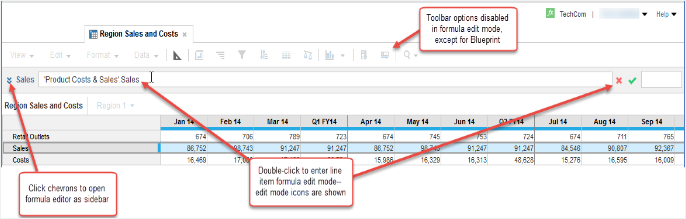If you have hyphens, numbers or any operator (+ - / *) in the line item text, make sure you include it in single quotes. If you are typing the formula, include quotes around each module and line item name—Anaplan will remove the ones it does not need.

For example: `Result = 'Ex-gratia payments'`.

A line item formula can be restricted so that it does not apply to all versions. You can set the Formula Scope for a line item formula with respect to model versions in Blueprint:

• All Versions
• Actual Version
• All Versions except Actual
• Current Version, which is designated in Settings, under Versions. Unless stated otherwise, the Current Version will be used if referred to by a line item formula, where the result does not have the Versions dimension applied.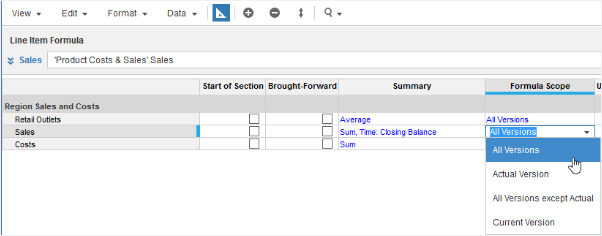The Summary selected for a line item determines the summary method used to calculate totals for the line item.

#### Adding Version formulas for line item subsets

This type of formula can apply to several line items at once for a given version. The formula scope is restricted to a single module.

1. To create the line item subset, in Model Settings > Lists and Roll-ups, click Line Item Subset and then click Insert.
2. Name the subset (for example, Regional Costs).
3. In your new subset, click in the module column to select the module you want to use. You can select Include New Items if you want to automatically expand the subset as items get added at a later date.
4.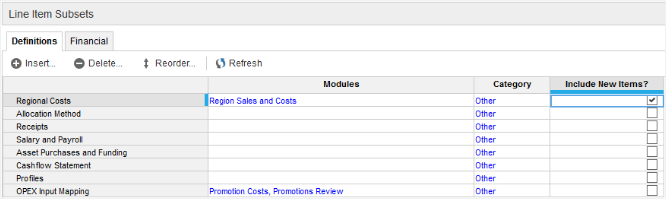1. Use the side menu to open your new subset and select the module items that you want to include in the line item subset:
2.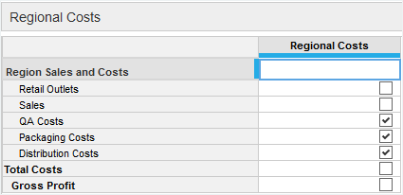3. In the module switch to Blueprint. On the Edit menu, click Add Version Formula then select the line item subset and version that the formula will apply to.
4.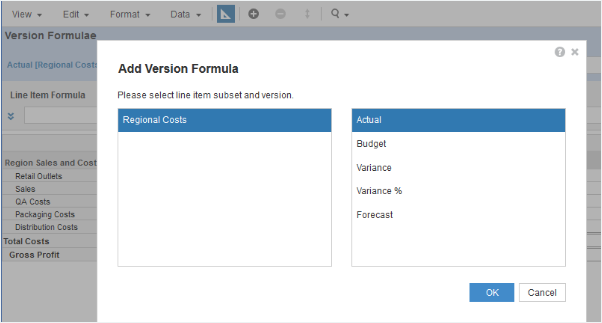A line item subset must exist to which at least one of the line items in the module belongs. If this condition is not met, then the left box on the dialog will be empty and you will not be able to create the module scope version formula.

5. Set up the formula in the version formula bar. This formula will apply across the subset of line items for the version chosen; it won't apply to other modules even if the line item subset spans several modules.
6.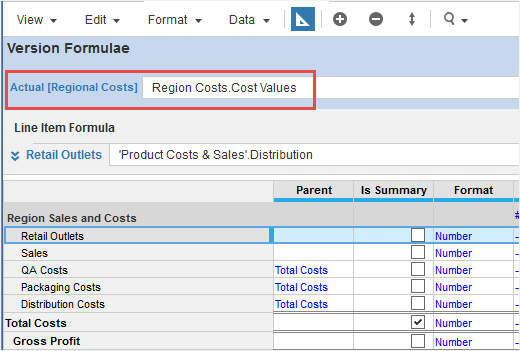If you select a line item in normal module grid view to which a module scope version formula applies, then this is shown in the formula bar. However, you can only edit the formula in Blueprint.

#### Adding Version Formulas for the Entire Model

This type of formula is typically used for variance calculations and spans the entire model, wherever the versions dimension is applied.

To set a versions formula, in Settings under Versions edit the formula directly by typing in the version formula in the Formula column: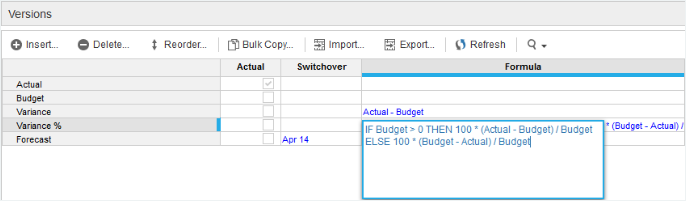#### Typical version formulas:

`Variance = Actual - Budget` `Variance % = 100*(IF Bud > 0 THEN 100 * (Actual - Bud) / Bud ELSE 100 * (Bud - Actual) / Bud)`

## Using calculation functions in a formula

Calculations use line item names, not obscure cell references.

A rich set of calculation functions is provided to match the modeling flexibility of Excel. Calculation syntax is consistent with Excel for familiar functions, such as conditional calculations.

Calculation functions include basic operators (+ - * / ^), bracketing, financial functions (such as depreciation and loan payments) and time and date functions.

Line items are linkable across modules. A line item in one module can be joined to another module or they can be related using conventional remote calculation references.

Powerful tools are provided to handle dimensional selections and transformations. For example, correspondences or mappings such as look-ups (one-to-many) or aggregations (many-to-one); allocations; and selective references (e.g. to refer to Total Costs for the IT department only). Complex qualified calculations with obscure syntax are avoided since a reference is divided into a basic item reference with any dimensional manipulation appended in a simple fashion.

Mappings may be based on dimension item properties, for example Product Pack Size, or on line item data, for example an assignment of Employee to Cost Center, which might for example vary by time.

The treatment mechanism provides for complex calculations applying to sets of line items. Financial Treatments handle calculations such as revenue recognition timings, debtors, impact on cash flow, that may apply to variable sets of line items. Currency conversion also uses the treatment mechanism to re-state monetary values in required currencies (according to the region and currency specified for each part of the organizational structure).

By default, calculations apply to all model versions: Current, Forecast, Actuals, and any prior forecasts included for comparison, but differing calculations may be specified for the Actuals version.

Case Sensitivity

If you're typing a formula, there's no need to use the correct case for line item names. For example:

`Sales = units * price `

will work even if the items are called Units and Price. The same applies to names of functions - you can type them in lower case and they will be reformatted to show in upper case.